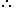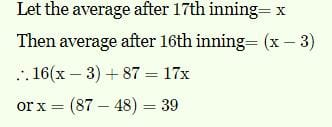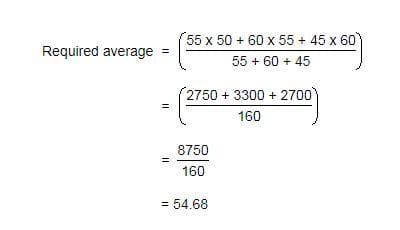Practice Test: Averages - 2

# Practice Test: Averages - 2

Test Description

## 10 Questions MCQ Test CSAT Preparation for UPSC CSE | Practice Test: Averages - 2

Practice Test: Averages - 2 for Quant 2022 is part of CSAT Preparation for UPSC CSE preparation. The Practice Test: Averages - 2 questions and answers have been prepared according to the Quant exam syllabus.The Practice Test: Averages - 2 MCQs are made for Quant 2022 Exam. Find important definitions, questions, notes, meanings, examples, exercises, MCQs and online tests for Practice Test: Averages - 2 below.
Solutions of Practice Test: Averages - 2 questions in English are available as part of our CSAT Preparation for UPSC CSE for Quant & Practice Test: Averages - 2 solutions in Hindi for CSAT Preparation for UPSC CSE course. Download more important topics, notes, lectures and mock test series for Quant Exam by signing up for free. Attempt Practice Test: Averages - 2 | 10 questions in 20 minutes | Mock test for Quant preparation | Free important questions MCQ to study CSAT Preparation for UPSC CSE for Quant Exam | Download free PDF with solutions
 1 Crore+ students have signed up on EduRev. Have you?
Practice Test: Averages - 2 - Question 1

### A grocer has a sale of Rs. 6435, Rs. 6927, Rs. 6855, Rs. 7230 and Rs. 6562 for 5 consecutive months. How much sale must he have in the sixth month so that he gets an average sale of Rs. 6500?

Detailed Solution for Practice Test: Averages - 2 - Question 1

Total sale for 5 months = Rs. (6435 + 6927 + 6855 + 7230 + 6562) = Rs. 34009.Required sale = Rs. [ (6500 x 6) - 34009 ]

= Rs. (39000 - 34009)

= Rs. 4991.

Practice Test: Averages - 2 - Question 2

### A batsman makes a score of 87 runs in the 17th inning and thus increases his averages by 3. What is his average after 17th inning?

Detailed Solution for Practice Test: Averages - 2 - Question 2Practice Test: Averages - 2 - Question 3

### Arun obtained 76, 65, 82, 67 and 85 marks (out in 100) in English, Mathematics, Chemistry, Biology and Physics. What is his average mark?

Detailed Solution for Practice Test: Averages - 2 - Question 3

Average = (76 + 65 + 82 + 67 + 85 )/ 5 = 375/5 =  75.

Practice Test: Averages - 2 - Question 4

If the average marks of three batches of 55, 60 and 45 students respectively is 50, 55, 60, what is the average marks of all the students?

Detailed Solution for Practice Test: Averages - 2 - Question 4Practice Test: Averages - 2 - Question 5

The average age of husband, wife and their child 3 years ago was 27 years and that of wife and the child 5 years ago was 20 years. What is the present age of the husband?

Detailed Solution for Practice Test: Averages - 2 - Question 5

Sum of the present ages of husband, wife and child = (27 * 3 + 3 * 3) years = 90 years.
Sum of the present ages of wife and child = (20 * 2 + 5 * 2) years = 50 years.
Husband's present age = (90 - 50) years
= 40 years

Practice Test: Averages - 2 - Question 6

The average weight of A, B and C is 45 kg. If the average weight of A and B be 40 kg and that of B and C be 43 kg, what is the weight of B?

Detailed Solution for Practice Test: Averages - 2 - Question 6

Let A, B, C represent their respective weights. Then, we have:

A + B + C = (45 x 3) = 135 .... (i)

A + B = (40 x 2) = 80 .... (ii)

B + C = (43 x 2) = 86 ....(iii)

Adding (ii) and (iii), we get: A + 2B + C = 166 .... (iv)

Subtracting (i) from (iv), we get : B = 31.B's weight = 31 kg.

Practice Test: Averages - 2 - Question 7

The average weight of 8 person's increases by 2.5 kg when a new person comes in place of one of them weighing 65 kg. What is the weight of the new person?

Detailed Solution for Practice Test: Averages - 2 - Question 7

The average weight of 8 persons increases by 2.5 kg
Total weight of 8 persons increased = 8 × 2.5 kg = 20 kg.
New person comes in place of one of them weighing 65 kg.
Hence the increase of weight of 8 persons together is because of new person replacing the person having 65 kg weight. Accordingly
Weight of new person = 65 + 20 kg = 85 kg
Thus the person replacing the 65 kg person should have 85 kg weight, which causes increase of weight of the group together by 2.5 kg .

Practice Test: Averages - 2 - Question 8

A student needed to find the arithmetic mean of the numbers 3, 11, 7, 9, 15, 13, 8, 19, 17, 21, 14 and x. He found the mean to be 12. What is the value of x?

Detailed Solution for Practice Test: Averages - 2 - Question 8

As, Mean = Total Sum of Observations / No. of Observations

Clearly, we have (3 + 11 + 7 + 9 + 15 + 13 + 8 + 19 + 17 + 21 + 14 + x) / 12 = 12.

or  137 + x = 144

⇒ x = 144 - 137 = 7.

Practice Test: Averages - 2 - Question 9

There are two divisions A and B of a class, consisting of 36 and 44 students respectively. If the average weight of divisions A is 40 kg and that of division b is 35 kg. What is the average weight of the whole class?

Practice Test: Averages - 2 - Question 10

Distance between two stations A and B is 778 km. A train covers the journey from A to B at 84 km per hour and returns back to A with a uniform speed of 56km per hour. Find the average speed of the train during the whole journey?

Detailed Solution for Practice Test: Averages - 2 - Question 10

Avg speed of train = 2xy/x+y
⇒ [2(84)(56)]/(84+56)
⇒ [2(84)(56)]/140
= 67.2km/hr

## CSAT Preparation for UPSC CSE

72 videos|64 docs|92 tests
 Use Code STAYHOME200 and get INR 200 additional OFF Use Coupon Code
Information about Practice Test: Averages - 2 Page
In this test you can find the Exam questions for Practice Test: Averages - 2 solved & explained in the simplest way possible. Besides giving Questions and answers for Practice Test: Averages - 2, EduRev gives you an ample number of Online tests for practice

## CSAT Preparation for UPSC CSE

72 videos|64 docs|92 tests